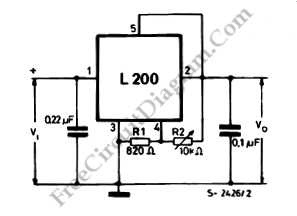# Programmable Voltage Regulator Using L200 ICThis is a programmable voltage regulator circuit. With this circuit we can generate a voltage according to our wishes. This circuit uses L200 IC. Here is the circuit :Potentiometer R2 is used to fix the output voltage Vo. Following equation show value of the output voltage:
Vo=Vref (1+(R2/R1))
We can obtain a wide range of fixed output voltage by substituting the potentiometer with a fixed resistor and choosing suitable values for R2 and R1. [Source: SGS-Thomson Application Note]